# SAT II Math I : Faces, Face Area, and Vertices

## Example Questions

### Example Question #1 : Faces, Face Area, And Vertices

How many vertices does a polyhedron with twenty faces and thirty edges have?Explanation:

By Euler's Formula, the relationship between the number of vertices, the number of faces, and the number of edges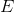of a polyhedron is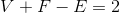Set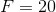and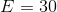, and solve for: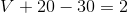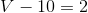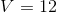The polyhedron has tweve vertices.

### Example Question #1 : Faces, Face Area, And Vertices

How many edges does a polyhedron with nine vertices and eleven faces have?Explanation:

By Euler's Formula, the relationship between the number of vertices, the number of faces, and the number of edgesof a polyhedron isSet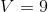and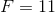and solve for: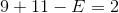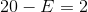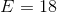The polyhedron has eighteen edges.